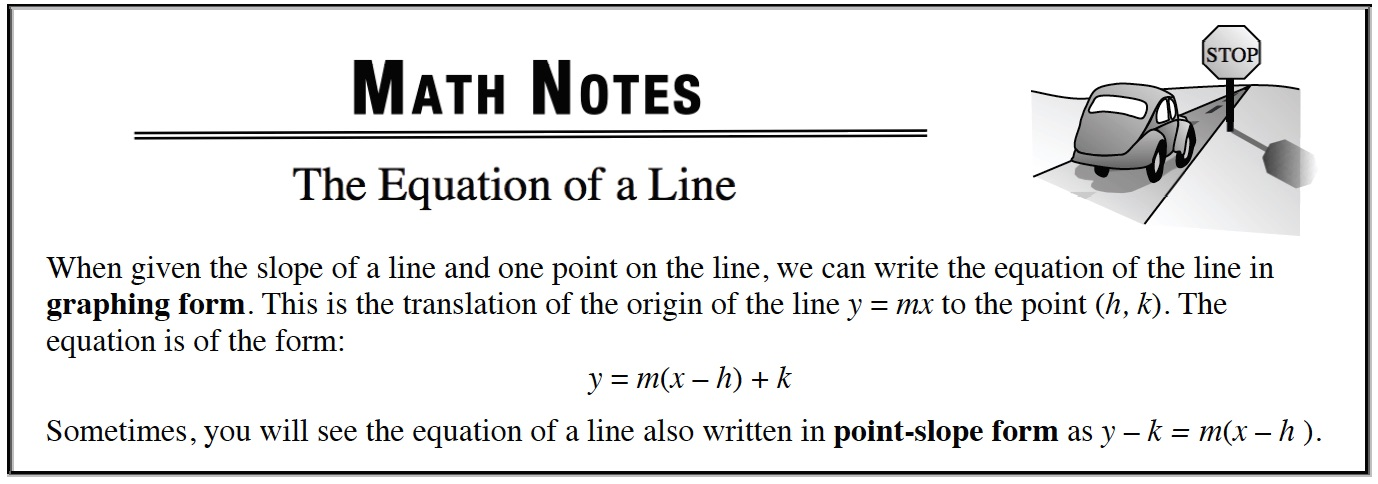### Home > APCALC > Chapter 1 > Lesson 1.4.2 > Problem1-156

1-156.

Write the equation of the line parallel to $9y - 4x = 12$ through the point $(6, –7)$. Write the equation in graphing form as shown in the Math Notes box preceding problem 1-8. Homework Help ✎

Since parallel lines have the same slope, find the slope by rearranging the equation so that $y =$ ____________.